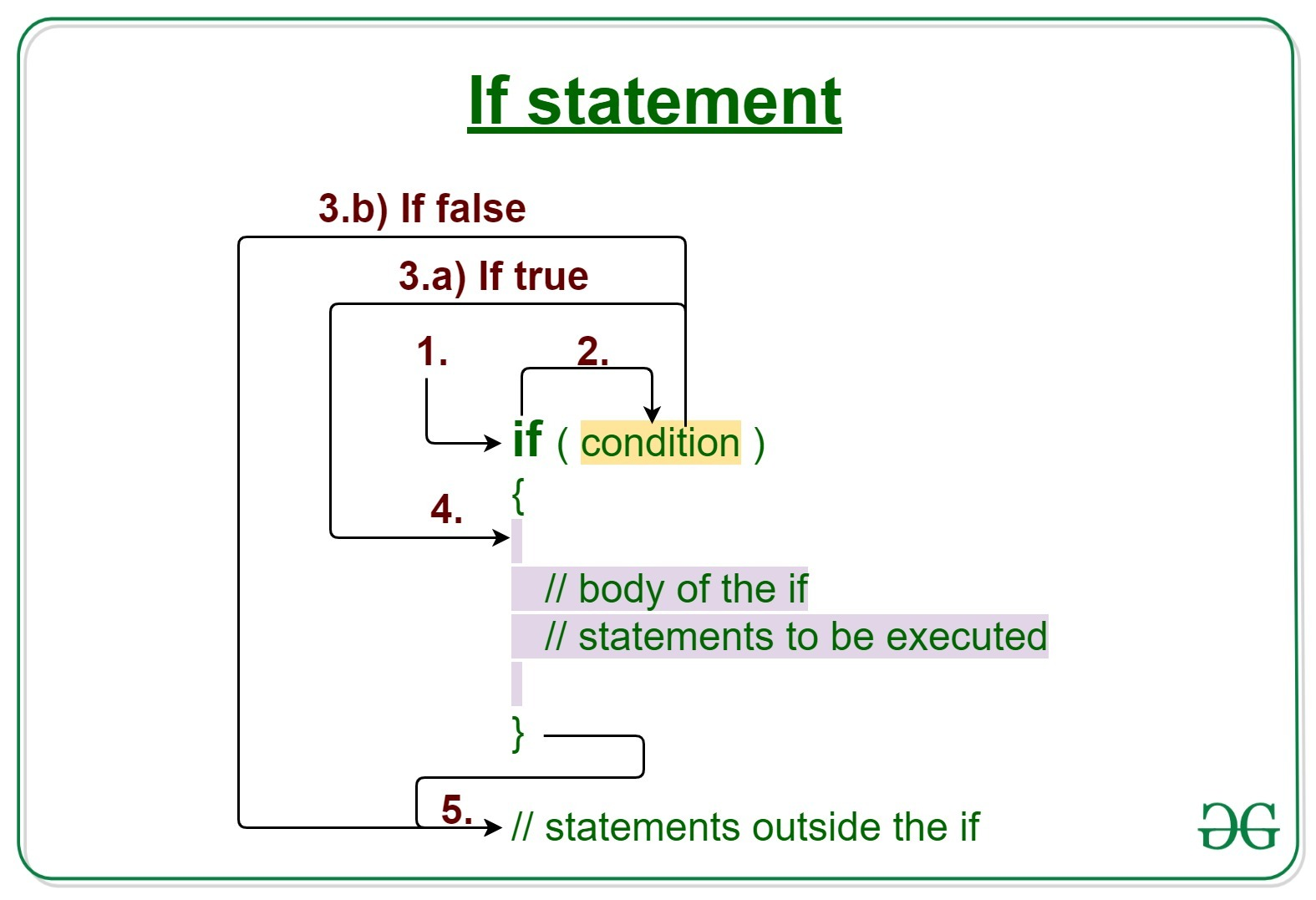# C/C++ if statement with Examples

Decision Making in C/C++ helps to write decision driven statements and execute a particular set of code based on certain conditions.

The C/C++ if statement is the most simple decision making statement. It is used to decide whether a certain statement or block of statements will be executed or not based on a certain type of condition.Syntax:

```if(condition)
{
// Statements to execute if
// condition is true
}```

Working of if statement

1. Control falls into the if block.
2. The flow jumps to Condition.
3. Condition is tested.
1. If Condition yields true, goto Step 4.
2. If Condition yields false, goto Step 5.
4. The if-block or the body inside the if is executed.
5. Flow steps out of the if block.

Note: If we do not provide the curly braces ‘{‘ and ‘}’ after if( condition ) then by default if statement will consider the immediate one statement to be inside its block. For example,

```if(condition)
statement1;
statement2;

// Here if the condition is true,
// if block will consider only statement1
// to be inside  its block.```

Example 1:

## C

 `// C program to illustrate If statement ` ` `  `#include ` ` `  `int` `main() ` `{ ` `    ``int` `i = 10; ` ` `  `    ``if` `(i < 15) { ` `        ``printf``(``"10 is less than 15 \n"``); ` `    ``} ` ` `  `    ``printf``(``"I am Not in if"``); ` `} `

## C++

 `// C++ program to illustrate If statement ` ` `  `#include ` `using` `namespace` `std; ` ` `  `int` `main() ` `{ ` `    ``int` `i = 10; ` ` `  `    ``if` `(i < 15) { ` `        ``cout << ``"10 is less than 15 \n"``; ` `    ``} ` ` `  `    ``cout << ``"I am Not in if"``; ` `} `

Output:

```10 is less than 15
I am Not in if
```

Dry-Running Example 1:

```1. Program starts.
2. i is initialized to 10.
3. if-condition is checked. 10 < 15, yields true.
3.a) "10 is less than 15" gets printed.
4. "I am Not in if" is printed.
```

Example 2:

## C

 `// C program to illustrate If statement ` ` `  `#include ` ` `  `int` `main() ` `{ ` `    ``int` `i = 10; ` ` `  `    ``if` `(i > 15) { ` `        ``printf``(``"10 is greater than 15 \n"``); ` `    ``} ` ` `  `    ``printf``(``"I am Not in if"``); ` `} `

## C++

 `// C++ program to illustrate If statement ` ` `  `#include ` `using` `namespace` `std; ` ` `  `int` `main() ` `{ ` `    ``int` `i = 10; ` ` `  `    ``if` `(i > 15) { ` `        ``cout << ``"10 is greater than 15 \n"``; ` `    ``} ` ` `  `    ``cout << ``"I am Not in if"``; ` `} `

Output:

```I am Not in if
```

Related Articles:

My Personal Notes arrow_drop_upCheck out this Author's contributed articles.

If you like GeeksforGeeks and would like to contribute, you can also write an article using contribute.geeksforgeeks.org or mail your article to contribute@geeksforgeeks.org. See your article appearing on the GeeksforGeeks main page and help other Geeks.

Please Improve this article if you find anything incorrect by clicking on the "Improve Article" button below.

Article Tags :
Practice Tags :

Be the First to upvote.

Please write to us at contribute@geeksforgeeks.org to report any issue with the above content.